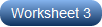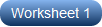1. Worksheets>
2. Math>
3. Geometry>
4. Angles>
5. Angles Around a Point

# Angles Around a Point Worksheets

Is "angles around a point" something your students are lagging behind in? Don't worry! Our print-ready, no-prep angles around a point worksheets, some of which are free, help teachers clearly explain the concept. Practice and review this concept with this great series of printable worksheets that focus specifically on finding the unknown angle around the center of the circle, finding the missing angle around a point, and finding the value of x when the missing angle is represented as simple expression. Equipped with answer key, each worksheet pdf facilitates instant verification of answers.

Find the unknown Angle: Easy

Enhance 4th grade and 5th grade students' engagement with these printable exercises that address finding the measure of the missing angle in a circle with its center as the common endpoint of the rays. As the angles around a point always add up to 360 degrees, figuring out the missing angle requires subtracting the measure of the known angle from 360.Find the unknown Angle: Moderate

Help students' practice become twice as effective with this set featuring measures of two or three angles around a point. All angles around a point sum up to 360 degrees. Use this fact to find the measure of the unknown angle by adding the known angles and subtracting the sum from 360 with these pdfs.Find the value of X

Using this bespoke resource for grade 4 and grade 5 children, you can check how well students know their angles around a point. The unknown angles are represented as simple expressions in terms of x. Form an expression by equating the sum of angles around a point to 360, and solve for x.Related Worksheets